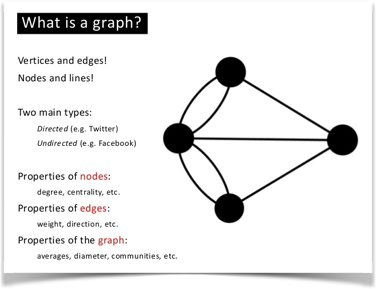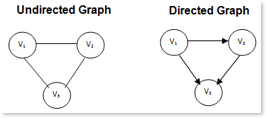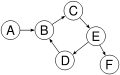# GraphStarting at the beginning means explaining what a graph is. A graph is mathematical concept which is used in computer science as a data structure, composed of nodes connected by edges. Each node represents some sort of entity, and each edge a relationship between two of those entities. Each edge may also have a direction and can store data. Social networks, such as Facebook, LinkedIn or Yammer, use this kind of data structure to represent the relationships between the people and the contents stored in it.

### Digraphs.A directed graph (or digraph) is a set of vertices and a collection of directed edges that each connects an ordered pair of vertices. We say that a directed edge points from the first vertex in the pair and points to the second vertex in the pair. We use the names 0 through V-1 for the vertices in a V-vertex graph.So, a graph is nothing more than a entity-relationship-sketch without the technical tricks. A graph can be easily rotated to provide a understandable access structure of the information. Applications and user experiences are setup this way. Boxary makes this explicit. In stead of targeting tables, matching where rules, the notation allows for specifying a path, matching attributes (compare pathfinders) and specifying the attributes to retrieve. To add intelligence a ''Lexicon' is provided.

Some rules must be considered:

• matching foreign keys must have the same attribute name.
• a relational table can be a vertex and a edge in the same time (refer to candidate keys).
• attribute names are unique.
• computed attributes are calculated from attributes of the very same vertex (relational table). Do not compute while traversing.

### Planar

A planar contains vertices and edges that constitute a particular model, which is used by the application. In general one could say that a Planar can be drawn on a planar, i.e. in 2D. Note that a single vertex is_a planar and a single edge is_a planar as well. This makes a Planar the base class, whilst it can describe structures consisting of more than one vertex / edge pair(s).